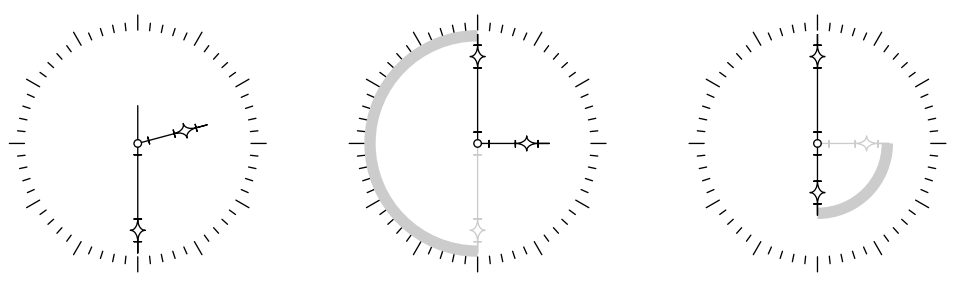시간 제한 메모리 제한 제출 정답 맞은 사람 정답 비율
2 초 128 MB 0 0 0 0.000%

## 문제

One famous Russian architect plans to build a new monumental construction. It will be a huge clock that indicates the time from the beginning of the universe.

The face of this clock contains hands, moving at constant speeds. They are numbered from 1 to n from the fastest to the slowest one. The fastest hand makes one revolution per minute (60 seconds). Each next hand moves slower than previous, the (i + 1)-th hand makes one revolution when the i-th hand makes di revolutions.

The setting mechanism of this clock is very simple. You can take a hand by the handle, located on its end, and move it in any direction. When you move the hand, slower hands are moving in proportion to their usual speeds, and faster hands are not moving. Remember that hands are huge, so setting this clock is a hard job.

Consider an example with three hands: a second hand, a minute hand, and an hour hand. Their lengths are 5, 15 and 10 meters respectively. You want to set the clock from 2:30 to 6:00 (fig. 1). The easiest way to do it is to rotate the minute hand 180 clockwise, and then move the hour hand 90 clockwise. The total distance you moved the handles of the hands is approximately 62.83 meters.Fig. 1. Setting clock from 2:30 to 6:00.

Your task is to write a program that finds the way to set the clock that minimizes the total distance you have to move the handles.

## 입력

The first line of the input file contains one integer n — the number of hands (0 < n ≤ 50). The second line contains n − 1 integer numbers d1, d2, . . . , dn−1 (2 ≤ di ≤ 106). The third line contains n integer numbers l1, l2, . . . , ln (1 ≤ li ≤ 106) — lengths of clock hands. Next two lines contain two non-negative integer numbers (one number per line): time indicated by the clock and the actual time that should be set. Both times are measured in seconds from the beginning of the universe and are less than 263.

## 출력

Print the minimal possible total distance you have to move the handles. The answer must be precise to at least 4 digits after decimal point.

## 예제 입력 1

3
60 12
5 15 10
52200
453600


## 예제 출력 1

62.831853072